# How to use the RADIANS Function in Excel

The radius of a circle is one-half of the distance across its center which makes an angle equal to 3.14159 radians. A degree is equal to 180 RADIANS because a whole circle is 360 DEGREES and is equal to two pi radians.

1 degree = (?/ 180) = (3.14159/180) radians
The PI function in excel returns the value of which is approximately 3.14159...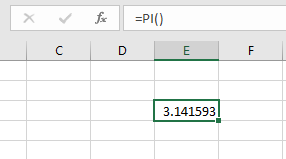The RADIANS function takes value of angle in degrees and returns the angle in radians.
Syntax:

angle : angle in degrees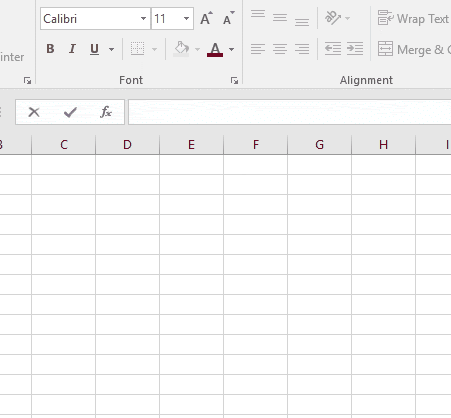As you can see, it proves the equation we stated above. The conversion of degree to radian is done using the RADIANS function in excel.
Now let’s get this function via running it on some examples.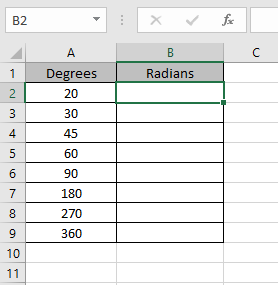Here we have some values as angle in radians and needs to get the angle in RADIANS
Use the formula: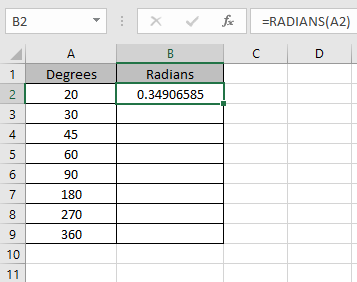20 degrees is approximately equal to 0.34906585.

Copy the formula using the shortcut key Ctrl + D.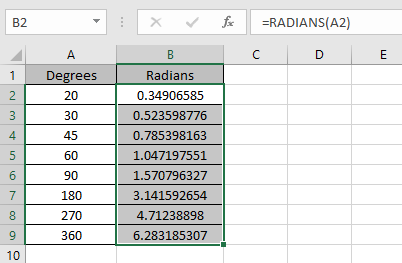As you can see from the above snapshot that angle in RADIANS can be extracted using the RADIANS function.
Hope you understood how to use RADIANS function and PI function in Excel. Explore more articles on Excel Date & Time functions here. Please feel free to state your query or feedback for the above article.

Related Articles:

How to use the DEGREES Function in Excel

How to use the Excel PI function

How to use SIN function in Excel

How to use the SINH Function in Excel

How to use the COSH Function in Excel

How to use the TANH Function in Excel

Popular Articles:

50 Excel Shortcuts to Increase Your Productivity

How to use the VLOOKUP Function in Excel

How to use the COUNTIF in Excel 2016

How to use the SUMIF Function in Excel

Terms and Conditions of use

The applications/code on this site are distributed as is and without warranties or liability. In no event shall the owner of the copyrights, or the authors of the applications/code be liable for any loss of profit, any problems or any damage resulting from the use or evaluation of the applications/code.